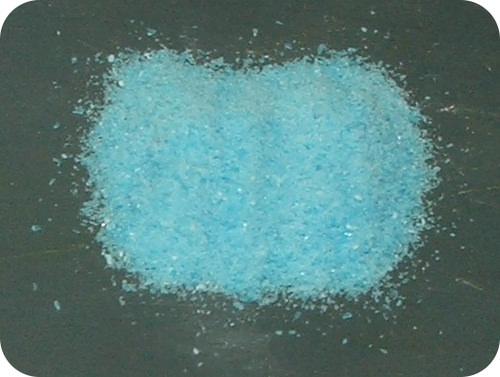# 10.11: Percent of Water in a Hydrate

$$\newcommand{\vecs}{\overset { \rightharpoonup} {\mathbf{#1}} }$$ $$\newcommand{\vecd}{\overset{-\!-\!\rightharpoonup}{\vphantom{a}\smash {#1}}}$$$$\newcommand{\id}{\mathrm{id}}$$ $$\newcommand{\Span}{\mathrm{span}}$$ $$\newcommand{\kernel}{\mathrm{null}\,}$$ $$\newcommand{\range}{\mathrm{range}\,}$$ $$\newcommand{\RealPart}{\mathrm{Re}}$$ $$\newcommand{\ImaginaryPart}{\mathrm{Im}}$$ $$\newcommand{\Argument}{\mathrm{Arg}}$$ $$\newcommand{\norm}{\| #1 \|}$$ $$\newcommand{\inner}{\langle #1, #2 \rangle}$$ $$\newcommand{\Span}{\mathrm{span}}$$ $$\newcommand{\id}{\mathrm{id}}$$ $$\newcommand{\Span}{\mathrm{span}}$$ $$\newcommand{\kernel}{\mathrm{null}\,}$$ $$\newcommand{\range}{\mathrm{range}\,}$$ $$\newcommand{\RealPart}{\mathrm{Re}}$$ $$\newcommand{\ImaginaryPart}{\mathrm{Im}}$$ $$\newcommand{\Argument}{\mathrm{Arg}}$$ $$\newcommand{\norm}{\| #1 \|}$$ $$\newcommand{\inner}{\langle #1, #2 \rangle}$$ $$\newcommand{\Span}{\mathrm{span}}$$$$\newcommand{\AA}{\unicode[.8,0]{x212B}}$$Figure $$\PageIndex{1}$$ (Credit: Doug Smith (Wikimedia: Xsmith); Source: http://commons.wikimedia.org/wiki/File:CurrituckSoundMap.png(opens in new window); License: Public Domain)

### Why does the color change?

If you look at a typical bottle of copper sulfate, it will be a bluish-green. If someone were to tell you that copper sulfate is white, you likely would not believe them. However, it turns out that you are both right; it just depends on the copper sulfate. Your blue-green copper sulfate has several water molecules attached to it, while your friend's copper sulfate is anhydrous (no water attached). Why the difference? The water molecules interact with some of the $$d$$ electrons in the copper ion and produce the color. When the water is removed, the electron configuration changes and the color disappears.

## Percent of Water in a Hydrate

Many ionic compounds naturally contain water as part of the crystal lattice structure. A hydrate is a compound that has one or more water molecules bound to each formula unit. Ionic compounds that contain a transition metal are often highly colored. Interestingly, it is common for the hydrated form of a compound to be of a different color than the anhydrous form, which has no water in its structure. A hydrate can usually be converted to the anhydrous compound by heating. For example, the anhydrous compound cobalt (II) chloride is blue, while the hydrate is a distinctive magenta color.Figure $$\PageIndex{2}$$: On the left is anhydrous cobalt (II) chloride, $$\ce{CoCl_2}$$. On the right is the hydrated form of the compound called cobalt (II) chloride hexahydrate, $$\ce{CoCl_2} \cdot 6 \ce{H_2O}$$. (Credit: (A) Martin Walker (Wikimedia: Walkerma); (B) Ben Mills (Wikimedia: Benjah-bmm27); Source: (A) http://commons.wikimedia.org/wiki/File:Cobalt%2528II%2529_chloride.jpg(opens in new window); (B) http://commons.wikimedia.org/wiki/File:Cobalt%2528II%2529-chloride-hexahydrate-sample.jpg(opens in new window); License: Public Domain)

The hydrated form of cobalt (II) chloride contains six water molecules in each formula unit. The name of the compound is cobalt (II) chloride hexahydrate and its formula is $$\ce{CoCl_2} \cdot 6 \ce{H_2O}$$. The formula for water is set apart at the end of the formula with a dot, followed by a coefficient that represents the number of water molecules per formula unit.

It is useful to know the percent of water contained within a hydrate. The sample problem below demonstrates the procedure.

##### Example $$\PageIndex{1}$$: Percent of Water in a Hydrate

Find the percent water in cobalt (II) chloride hexahydrate, $$\ce{CoCl_2} \cdot 6 \ce{H_2O}$$.

###### Solution

Step 1: List the known quantities and plan the problem.

The mass of water in the hydrate is the coefficient (6) multiplied by the molar mass of $$\ce{H_2O}$$. The molar mass of the hydrate is the molar mass of the $$\ce{CoCl_2}$$ plus the mass of water.

###### Known
• Mass of $$\ce{H_2O}$$ in $$1 \: \text{mol}$$ hydrate $$= 108.12 \: \text{g}$$
• Molar mass of hydrate $$= 237.95 \: \text{g/mol}$$
###### Unknown
• percent H2O = ?%

Calculate the percent by mass of water by dividing the mass of $$\ce{H_2O}$$ in 1 mole of the hydrate by the molar mass of the hydrate and multiplying by $$100\%$$.

###### Step 2: Calculate.

$\% \: \ce{H_2O} = \frac{108.12 \: \text{g} \: \ce{H_2O}}{237.95 \: \text{g}} \times 100\% = 45.44\% \: \ce{H_2O}\nonumber$

Nearly half of the mass of the hydrate is composed of water molecules within the crystal.

## Summary

• The process of calculating the percent water in a hydrate is described.

## Review

1. What is a hydrate?
2. How can you convert a hydrate to an anhydrous compound?
3. What does hexahydrate mean?

This page titled 10.11: Percent of Water in a Hydrate is shared under a CK-12 license and was authored, remixed, and/or curated by CK-12 Foundation via source content that was edited to the style and standards of the LibreTexts platform; a detailed edit history is available upon request.